### Casino Probability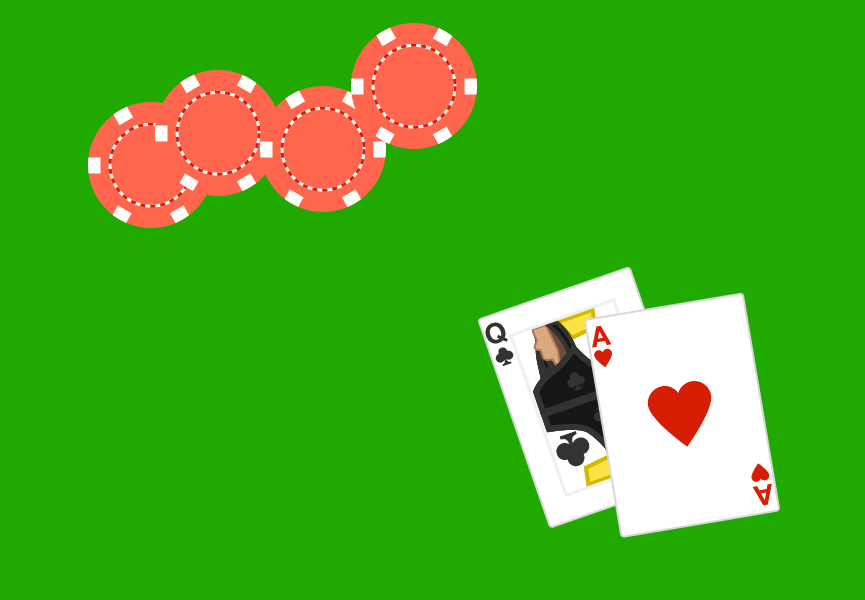Welcome to Casino Games! This course focuses on learning the probabilities behind Blackjack, Craps, and Poker. While it can help to have some background (with our Probability Fundamentals course), the material here is self-contained and can be done on its own.

For these introduction quizzes, we'll warm up with some questions on cards and dice, and then dive into analyzing two games that have been in real casinos.

# Cards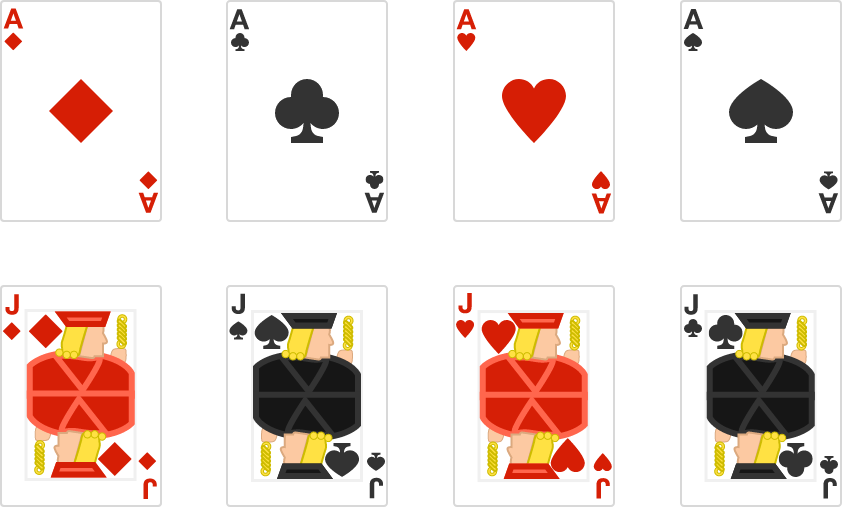If you draw one card at random from the $8$ shown above, what is the probability you draw a Jack or a Diamond (or the card that is both)?

# Cards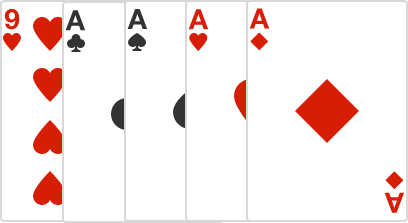If you draw four cards at random (without replacement) from the five shown above, what is the probability all four cards are aces?

# Cards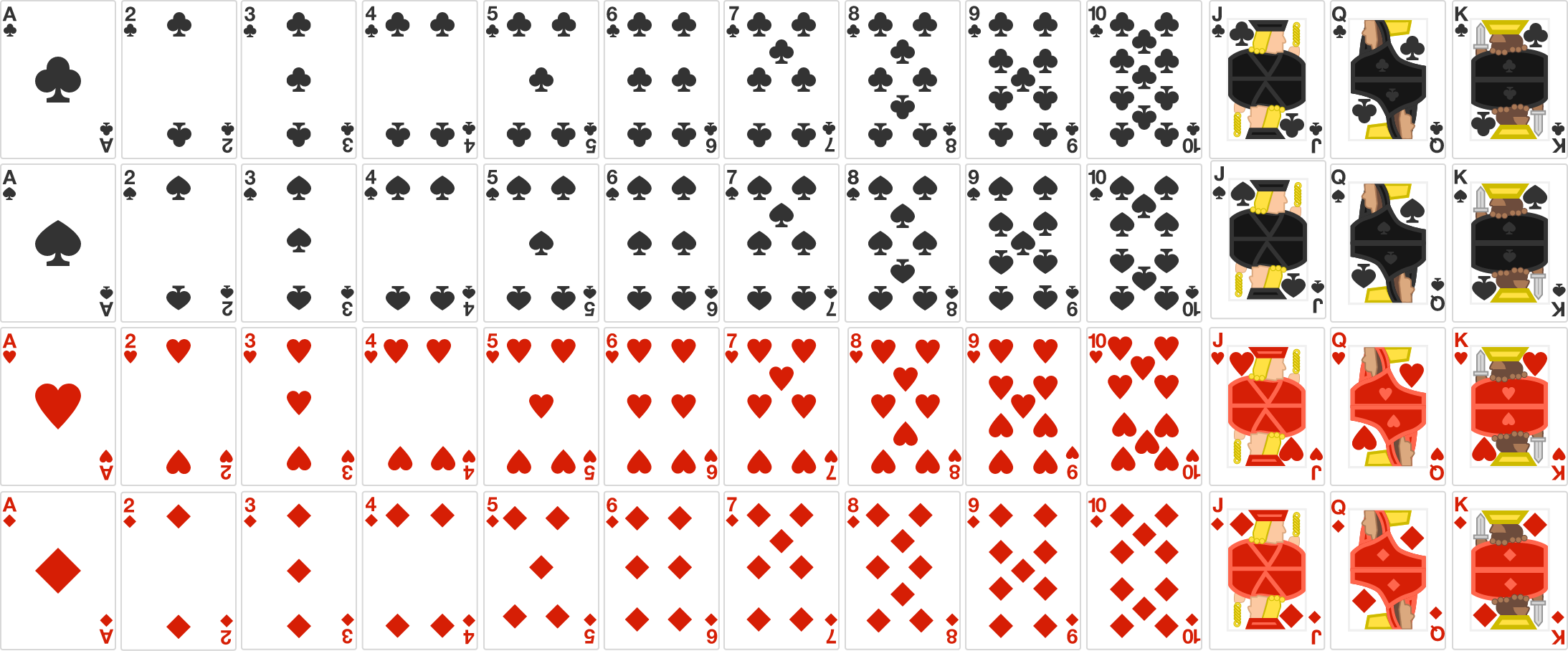If you draw $2$ cards (without replacement) from a shuffled standard $52$-card deck, what is the probability they are the same suit?

$($The suits are hearts, clubs, diamonds, and spades, and there $13$ of each type$.)$

# Cards

Amy and Blake are each dealt two cards from the same standard $52$-card deck. Amy's cards are known, while Blake's cards are unknown. In which of these two scenarios is it more likely that Blake has a pair?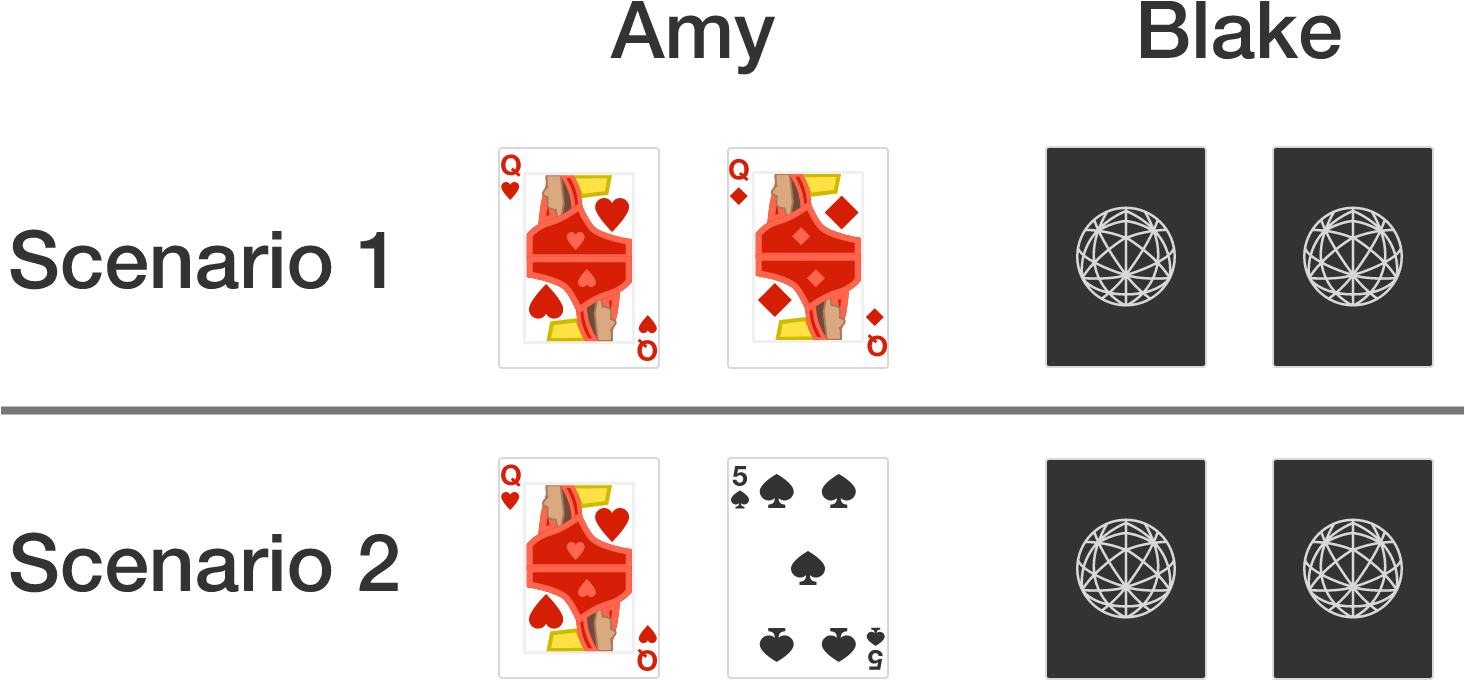$($In poker, a pair involves two cards of the same face value $(2, 3, 4, \ldots , \text{Q}, \text{K}, \text{A}),$ not the same suit$.)$

# Cards

With casino games, working out the best strategy isn't just a matter of probabilities but how much money you earn for each bet!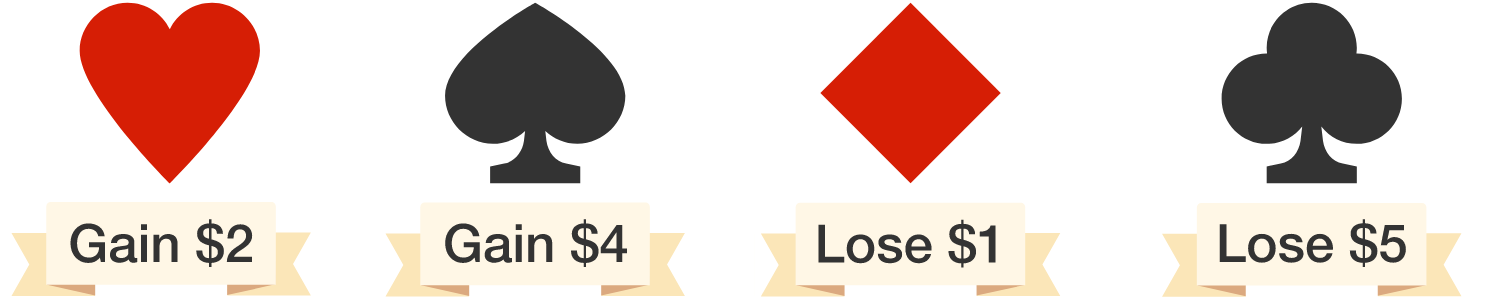Suppose you draw cards at random from a standard $52$-card deck, and for each card you either earn or lose money based on the suit drawn (as shown above).

Is this a fair game (that is, on average, do you gain as much money as you lose)?

×